# 5 Airtable IF-Statements to Supercharge your Workflow

by Juliet Edjere · December 2021
5 Airtable IF-Statements to Supercharge your Workflow
5 Airtable IF-Statements to Supercharge your Workflow

Contents

In this article, we will highlight 5 IF-Statements to automatically generate information from your tables. Airtable is an online collaboration platform that works like a spreadsheet and a database. The low-code platform allows you to customize your workflow and integrate with 300+ apps and services.

As a relational database, Airtable is designed to represent the relationships between different concepts and/or objects. You can link two tables together by using formulas that refer to values in other cells.

Using formulas in Airtable is quite similar to Excel, but with some key differences: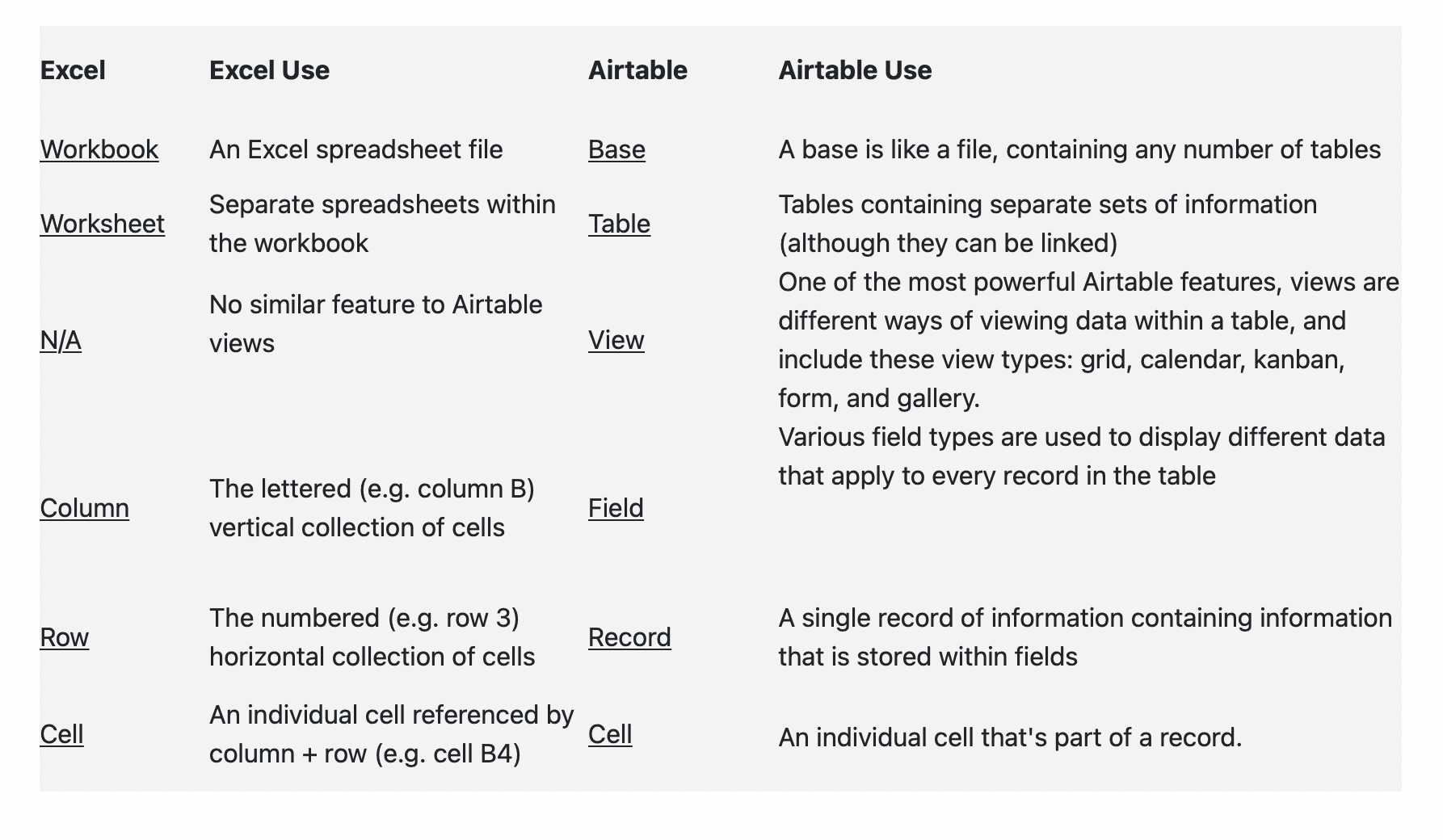Formulas help to make large amounts of data simple. The value can be words or numbers (calculation). If you're new to formulas, Airtable has an article on formula writing tips for beginners.

## What are IF-statements?

‘If-statements’ are used to perform a logical test to evaluate whether a condition is true or not. It returns a defined value if the condition is true, and a different value if it’s false.

To display whether something is true or not, add a formula field to your table and enter the ‘if-statement’:

The syntax of IF-THEN is **`IF**(logical test, value if true, [value if false])`

The first argument tells the function what to do if the comparison is true. The second argument tells the function what to do if the comparison is false.

For instance, IF(Sales > 50, "Win", "Lose") returns Win if the logical argument is greater than 50, otherwise, it returns Lose.

## Basic True and False Arguments

The basic example of an IF statement validates if a statement is true or false.

``````IF({Test Score} < 80, "Fail", "Pass")
``````

Use curly brackets to reference field names with more than one single word or character. In this case, {Test Score} has spaces in its field name so it's a best practice to surround the field name with curly brackets when referencing the field.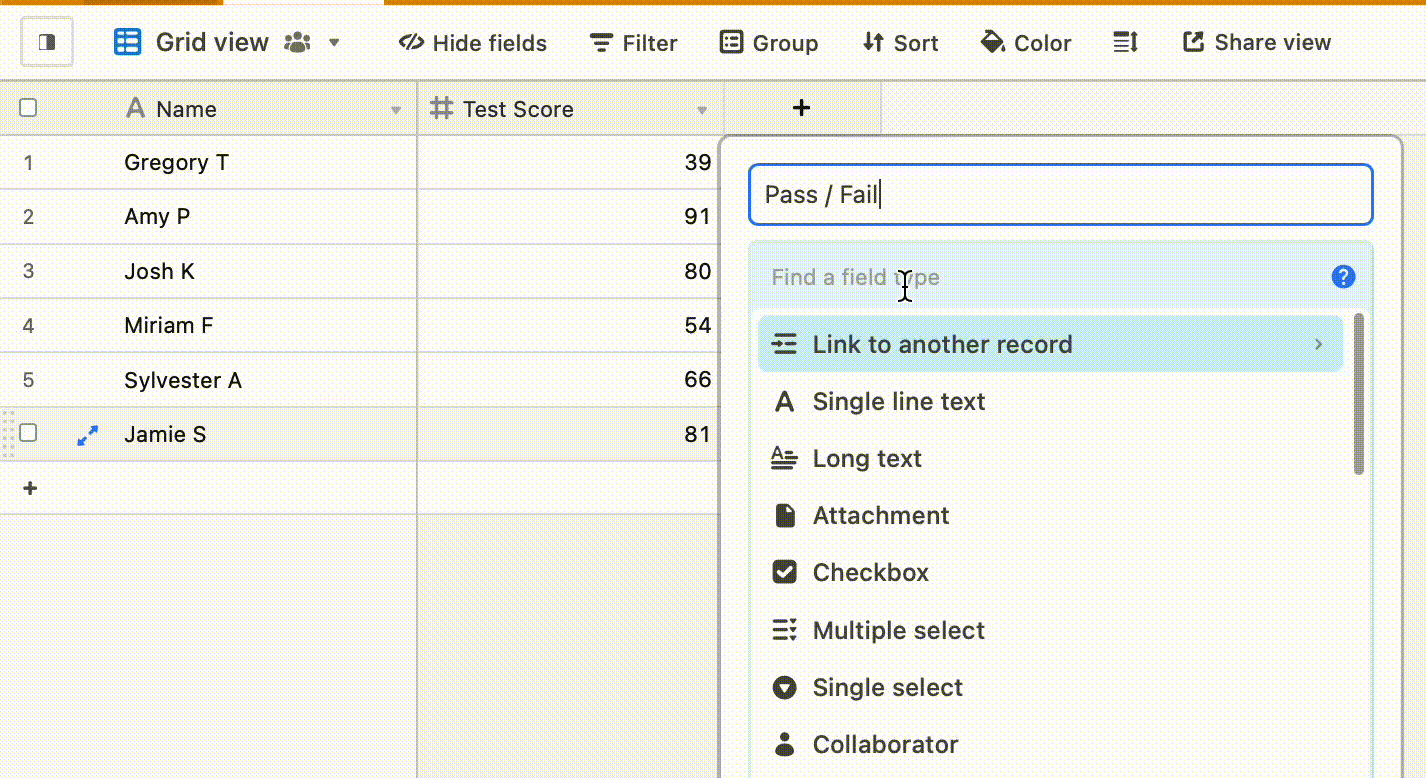In this case, we are testing to see if the test score is less than 80. The formula will return “Fail” if the first argument is true but return “Pass” if the second argument is false (i.e. is not less than 80).

## Perform a Calculation

Instead of a statement, an IF statement can calculate numbers between fields. You can perform a calculation within the logical_test or the values sections.

``````IF(100 > {Test Score}, 100 - {Test Score}, "")
``````

In this statement, the formula field subtracts one value from another.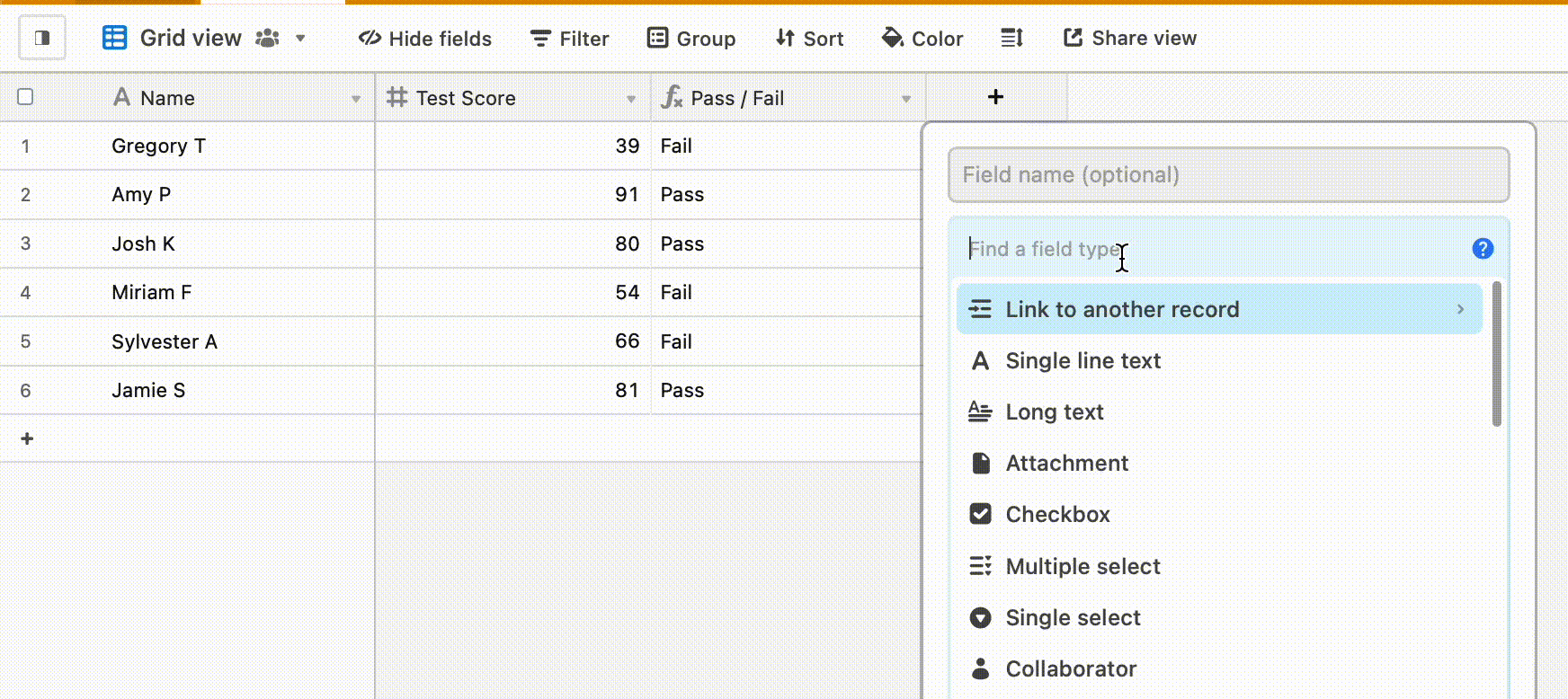So, if the argument is true that 100 is greater than the test score, the difference will be the result. If it is false, the field returns no value since the value_if_false is omitted.

## Test If a Date Is Today

An ‘if-statement’ can evaluate if a date provided matches today's date based on the value of a date field.

``````IF(Date = TODAY(), "True", "")
``````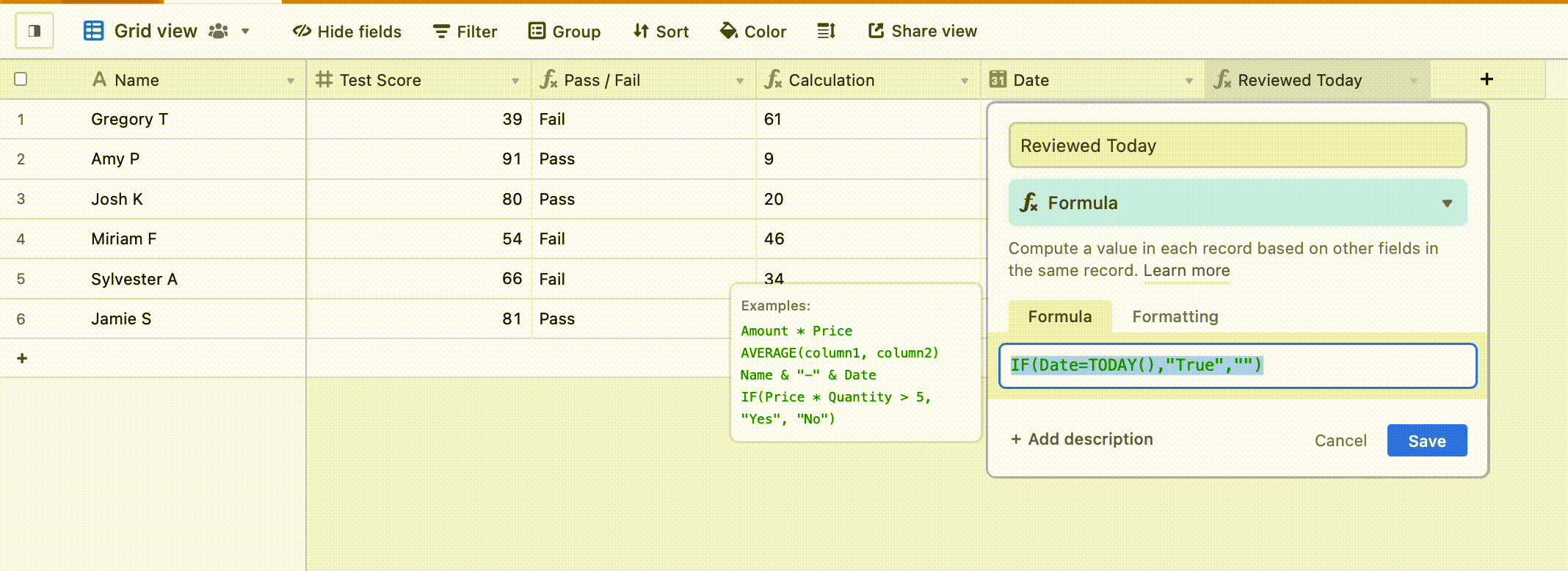For fields with no spaces in the field name (e.g. Date), you don't have to add curly brackets around the field name. Based on best practices, we recommend that you add curly brackets.

## Using AND() with IF Formula

AND(logical1, [logical2, …]) returns true if all the arguments are true, return false otherwise.

The entire formula will be structured using an IF() function, even though it will have the AND() function within it.

The AND() function sits within the first set of commas, thus it is the IF logical_test:

``````IF(
AND(
{Status} = "Not Started"
),
""
)
``````

If the deadline is within 30 days from now, and the status is not started, then show an alert, otherwise show nothing.

DATETIME_DIFF(From, To, units) returns the difference between datetimes in specified units.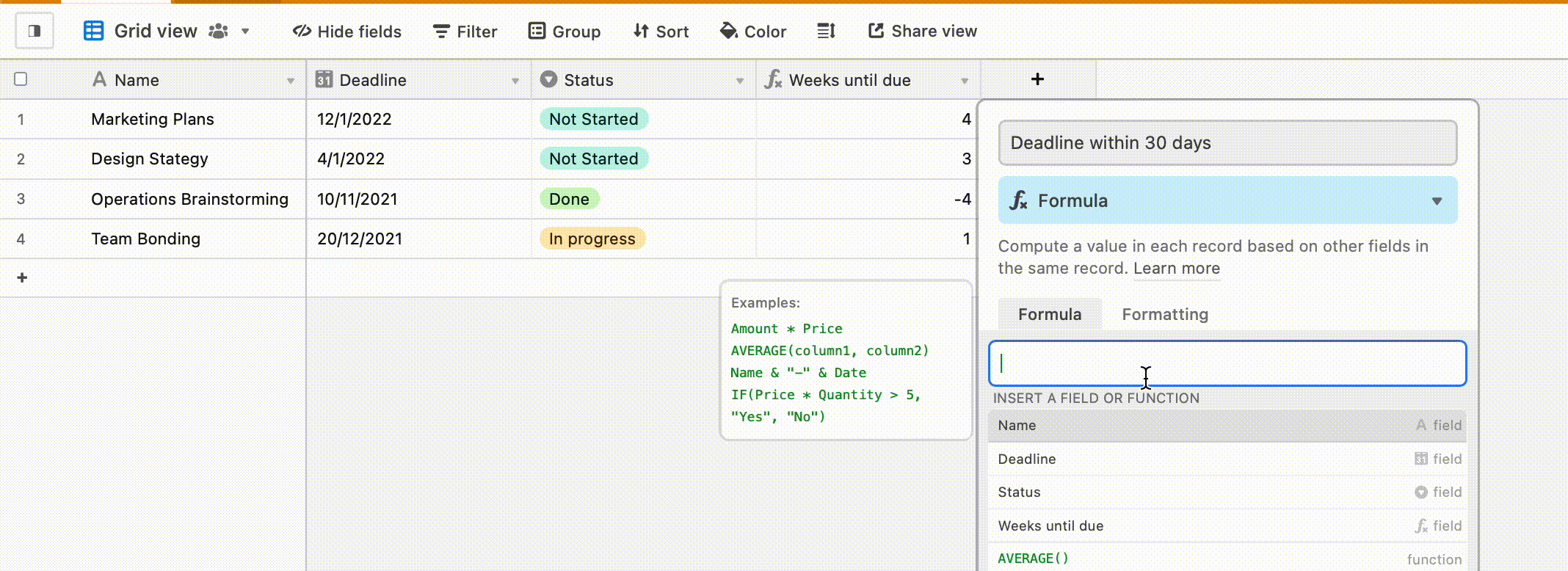The AND() function specifies that the field should show Alert! only IF the deadline is within 30 days from now AND the status is not started.

## Nested IF statements

When more than one IF statement is included in the formula, this is called a Nested IF statement. These are conditional-heavy formulas.

With nested statements, all of the parentheses used to separate out containers for the formula function must always contain opening and closing parentheses.

We’ll work with a formula that tells us the phase a project is in based on how close the deadline is.

We created a field called “Weeks until due” that provides us with the number of weeks until the deadline. This field is created with the DATETIME_DIFF formula.

``````DATETIME_DIFF({Deadline}, TODAY(), 'weeks')
``````

Depending on the weeks until due, we want the formula field to assign phases to each record based on If the deadline is:

• 4 weeks until due = planning phase
• 3 weeks until due = execution phase
• 2 weeks until due = review phase
• 1 week until due = launch phase
• Less than = out of range

IF( {Weeks until due} = 4, 'Planning', IF( {Weeks until due} = 3, 'Execution', IF( {Weeks until due} = 2, 'Review', IF( {Weeks until due} = 1, 'Launch', IF( {Weeks until due} < 1, 'Out of range' ) ) ) ) )

The number of parentheses you need at the end of a formula with nested IF functions is the same number of times IF is written.

Using the Nested IF() statement results in a different project phase depending on the {Weeks until due} field.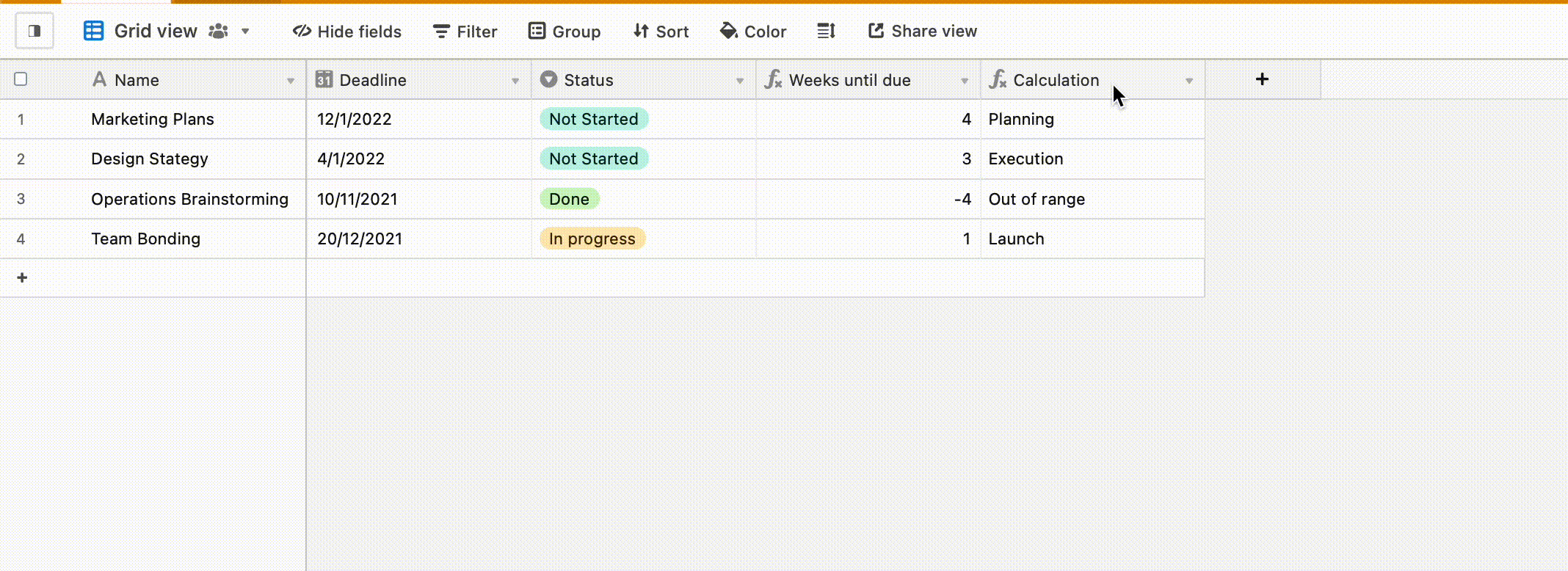A cleaner alternative to nested IF statements is using the SWITCH() statement which removes the need for nesting.

SWITCH(expression [, pattern, result]… [, default]) matches the expression against a series of pattern values, returning the result value corresponding to the first match. Pattern values must be unique. If no patterns match, returns the default value if specified, otherwise null.

``````SWITCH(
{Weeks until due},
4, 'Planning',
3, 'Execution',
2, 'Review',
1, 'Launch',
'Out of range'
)
``````

## Key Takeaways

These 5 formulas can serve as building blocks for more complex formulas. It is possible to nest multiple IF functions within one formula.

There aren't any defined limits on how long a formula can be, or how many IF statements can be included.

The strategy for writing complex formulas is to first write the problem out in plain language. Then, break down the problem into a series of logical arguments that have only one operator. With this strategy, it becomes easier to nest three or more IF statements.

You can nest a series of IF functions to create a complex IF THEN ELSE statement that can return multiple possible outcomes.

## Related Posts

Other resources on Bannerbear with the tags nocode and airtable